## Jan 8, 2015

### Raymarching a Christmas Tree

Here's a GLSL fragment shader code:

```                                                          float

v(vec3 v,

float y){return

v.y+y;} float f(vec3

v,float y){return v.z+y;}

float n(vec3 v,float y){return

-v.z+y;} float s(vec3 v,float y){return

length(v)-y;} float f (in vec3 v,float y, float i)

{return max(abs(v.y)-i, length(v.xz))- y*clamp(i-abs(v

.y),0.,i);} float i(vec3 v,vec2 y){vec2 i=abs(vec2(length

(v.xz),v.y))-y;return min(max(i.x,i.y),0.)+length(max(i, 0.));}

float m(vec3 v,vec2 y){vec2 i=vec2(length (v.xy)-y.x,v.z);return length

(i)-y.y;}  float p(float v,float y) {return max(-y,v);} float e(float v,float

y) {return min(y,v);} vec2 c(vec2 v,vec2 y){return v.x<y.x?v:y;} float r(float v,

float y) {return max(y,v);} vec2 x(vec2 v,float y) { float i= cos(y),f = sin(y); return

vec2(v.x*i- v.y*f,v.x*f+v.y*i); } float h(float v,float y) { return mod(v,y)-y*.5; } vec3 t

(vec2 v,float y) { float i=6.28319/y; vec2 f=x(v,-i*.5); float z=floor(atan(f.x,f.y) /i);v=x(v,z*i);

return vec3(v.x,v.y,z);} float c(vec3 v) { return v.xy= t(v.xy,5.).xy, v.y=v.y-.3,v.xz=abs (v.xz),

dot(v,normalize(vec3 (2.,1,3.)))/3.;} float d(in vec3 v,float y) { float i=s(v,.5); if(y<= 0.) i+=

cos(atan(v.x,v.z)*30.)*.01*(.5-v.y) +sin(v.y*60.)*.01; else if(y<=1.) i=p(i,s(v+vec3 (0.,0.,-.9),.7));

else if(y<=2.) return i+=cos(v.y*28.)

*.01; else if(y<=3.) i+=cos(atan(v.x,v.z)*20.)*.01*(.5-v.y);

return i; } vec2 d(in vec3 v) { return v.y+=(sin(sin(v.z*.253)+v.x

*.11)*.31+cos(v.z*.53+sin(v.x*.27))*.12)*1.2+.2,vec2(v.y,1.); } vec2 e

(in vec3 y) { vec3 f=y; float z=abs(-floor(f.y/1.9)/4.+1.)*3.; vec3 n=t(f.xz,

2.7*z); float x=z*113.+n.z*7.+55.; f.y+=mod(x,17.)*.03-.4;f.xz=n.xy; f.y=mod(f.y,1.9)

-.5; f+=vec3(0,0,-z); float l=mod(x,5.),s=d(f,l),a=i(f-vec3(0.,.5,0.),vec2(.08,.1)); a=e(a,m

(f-vec3(0.,.62,0.),vec2(.05,.015)));vec2 k=c(vec2(s,x),vec2(a,3.)); k.x=r(k.x,v(y,-8.)); return k;

} vec2 f(in vec3 v) { v.y-=8.3; v*=.5; float y=e(c(v),i(v-vec3(0.,-.2,0.),vec2 (.04,.1))); return vec2

(y,2.);} vec2 h (in vec3 v){vec3 y=vec3(v); float i=length(y.xy); y.xy=x(y.xy,-i*.5); y.z=h(y.z,.25);y.xy=

t(y.xy,17.).xy; y.yz=x(y.yz,(.15-i)*1.4+y.x);float z=f(y,.05,.5);z=r(z,f(v,0.)); float m=r(i-.02,f(v,.125));return

c(vec2(z,4.),vec2(m,5.));} vec2 i(vec3 v) { v.y+=.204; float y=floor(v.y/1.7),i=max(2.,9.-y*1.2);v.xz=t(v.xz,i).xy; v.z

-=3.81; v.yz=x(v.yz,.423); v.y=h(v.y,1.7); return h(v); } vec2 m(vec3 v) { vec2 y=i(v); v.xz=x(v.xz,.53); v.y-=.68; y=c(

y,i(v)); vec2 z=vec2(f(v.xyz,.02,8.),5.); y=c(y,z); y.x=r(y.x, s(v-vec3(0.,4.,0.),5.7)); return y; } vec2 n(in vec3 v) {

vec2 y=d(v); y=c(y,f(v)); y=c(y,e(v)); y=c(y, m(v)); return y;} vec3 p(in vec3 v) { vec2 y=vec2(.001,0.); return normalize

(vec3(n(v+y.xyy).x-n(v-y.xyy).x, n(v+y.yxy).x -n(v-y.yxy).x,n(v+y.yyx).x -n(v-y.yyx).x));} vec2 l(in vec3 v,in vec3 y) {

float i=1.,f=-1.; for(int  z=0;z<256;z++)

{ vec2 m=n(v+y*i); if(m.x<.001||i>100.) break;

i+=m.x; f=m.y; } if(i>100.) f=-1.; return vec2(i,f);} vec3

a(vec3 v,float y) { return mix(v,vec3(.3,.342,.5),1.-exp(-.001*y*y));} vec3

a(float v) { vec3 y=vec3(.3,.342,.5); if(v<=1.) y=vec3 (3.3,3.3,4.5); else if(v<=2.)

y=vec3(1.6,1.,.6); else if(v<=3.) y=vec3(1.2,1.,.8); else if(v<=4.) y=vec3 (.152,.36,.18); else if(v

<=5.) y=vec3(.79,.51,.066); else y=.3+.7*sin(vec3(.7,.4,.41)*(v-6.)); return y;} float a(vec3 v,vec3 y,

float i,float z) { float f=1., r=i; for(int m=0;m<64;m++) { float x=n(v+ y*r).x; f=min(f,8.*x/r); r+=clamp(x,.02,

.2); if(x<.001||r>z) break; } return clamp(f,0.,1.);} vec3 g(vec3 v,in vec3 y) { vec3 i=vec3(0.); float f=1.;

for(float z=0.;z<3.;z++) { vec2 m=l(v,y); float x=m.x,s=m.y; vec3 c=v+x*y,r=p(c),n=reflect (y,r),e=a(s),d=normalize(

vec3(-1.2,.3,-1.1)); float t=clamp(dot(r,d),0.,1.),k=pow(clamp(dot(n,d), 0.,1.),10.); e*=a(c,d,.01,1.); e*=t*(vec3(.8,1.,

.9)+1.2*k*vec3(.8,.9,.6)); e=a(e,x); i+=e*f; if(s<=6.) break; v=c+n*.001; f*=.5; y=n; } return vec3(clamp (i,0.,1.));}

void main() { vec2 y=gl_FragCoord.xy/iResolution.xy, v=-1.+2.*y; v.x*= iResolution.x/iResolution.y; float i=40.+

iGlobalTime,f=.1*i; vec3 z=vec3(13.*cos(f),4.,13.*sin(f)), x=normalize(vec3(0,3.8,0)-z), r=vec3(0.,1.,0.),s=normalize

(cross(x,r)),m=normalize(cross(s, x))

, d = normalize( v.x *s + v.y*m + 2.5

*x),a=g(z,d); gl_FragColor=vec4(a,1.);}
```

which renders a ray-traced 3D scene: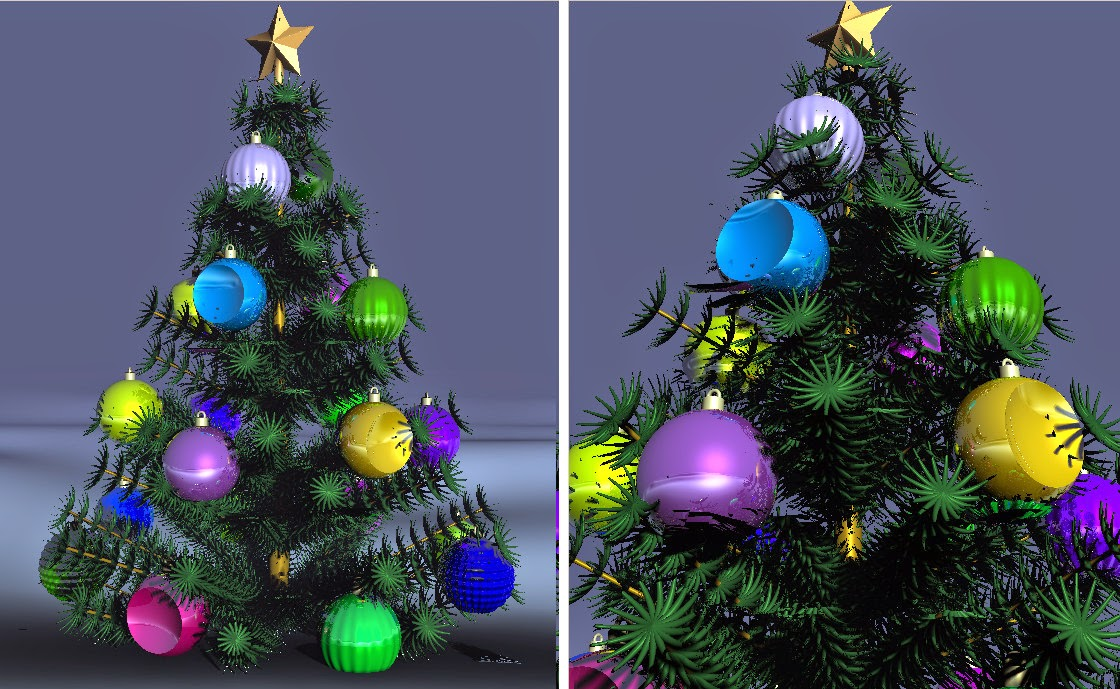(the non-obfuscated version is on GitHub, and the result can be seen online on ShaderToy. Be aware, that thing requires WebGL-enabled browser and a decent videocard).

No data input required, it's a self-contained code, which does both the procedural scene description and raytracing/shading, all in a single fragment shader. The only assumptions are that we draw a fullscreen quad (AKA "two triangles") and there are a couple of uniforms (time and resolution) externally set.

If you've seen the stuff people put on ShaderToy, and especially the amazing work of its creator Inigo Quilez, then it might not amuse you too much.

Otherwise, read on for the recipe.

### Ingredients

To cook a Christmas tree shader, such as this, you will need:

• A so-called "distance function", which in a sense is a way to describe the scene geometry (in our case, as one big math formula)
• Code that implements a "raymarching algorithm" (using the aforementioned distance function)
• A way to somehow specify material properties for different parts of the scene
• A shading code, which computes the color of a pixel according to some lighting model
• "Camera" code, which handles the current position and ray direction for the given fragment
• Spice up with a fog, reflections, shadows, ambient occlusion and other effects, to taste
• Optional: serve in the obfuscated form

### Distance function

Traditionally, 3D scenes are represented via polygonal (triangular) meshes, or some higher-level analytical description of the geometry.

But let's say, that instead of having an explicit scene description (polygonal or whatever), we somehow managed to specify a certain "magic" function. This function, for any point in space, would return a distance to the closest point (wherever it is) on some of the scene's objects:

``````//  returns the distance from the given point in space
//  to the closest point on the scene's geometry
float distf(vec3 pos) {
float d = ... // some computations
return d;
}
``````

We don't really need to know where exactly is this closest point located, just a distance to it.

Furthermore, we don't even need the exact distance - only a "good enough" upper boundary (i.e. some value, which is guaranteed to be not smaller that the real distance).

An important property is that the distance function is signed, meaning that if our point pos is inside some object, the returned distance will still make sense. However, it will be negative, and its absolute value is bigger or equal to the closest distance to get "outside".

### Raymarching algorithm

What's the use of such a function for the Christmas trees?.. Well, the already mentioned ray marching optimization is one of the benefits:(sorry, could not help myself)

Seriously, though, also known as a "sphere tracing", it's nothing else than just an optimization of the raytracing step.

The basic idea of raytracing is that for each pixel in the image plane we shoot the corresponding ray from the eye into the scene and find where this ray hits the geometry or light sources (possibly bouncing around).

Ideally, we should be able to analytically find this intersection point, given some scene representation: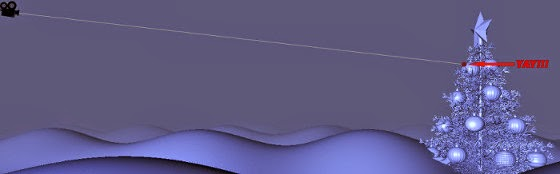This is feasible for the basic environments (e.g. composed from spheres), but in practice gets complicated, if not impossible, when more complex shapes are added.

So instead, we step along the ray in small increments, every time asking a question: "are we there yet?" (in other words, has the ray penetrated any object yet). That's what is called raymarching, and it obviously can be very inefficient for small step sizes: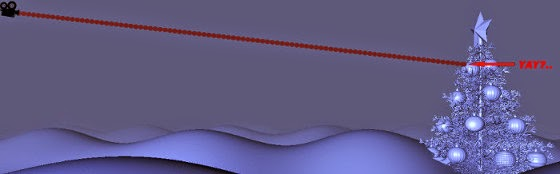When we have a distance function, however, we can potentially proceed in much bigger steps, since we know that there is no way we can penetrate any object, stepping by the amount returned from the distance function:The shader code that is preforming the described procedure:

``````
#define NEAR_CLIP_PLANE         1.0
#define FAR_CLIP_PLANE          100.0
#define MAX_RAYCAST_STEPS       200
#define STEP_DAMPING            0.7
#define DIST_EPSILON            0.001

float rayMarch(in vec3 rayOrig, in vec3 rayDir) {
float t = NEAR_CLIP_PLANE; // current distance along the ray
for (int i = 0; i < MAX_RAYCAST_STEPS; i++) {
float d = distf(rayOrig + rayDir*t);
if (d < DIST_EPSILON || t > FAR_CLIP_PLANE) break;
t += d*STEP_DAMPING;
}
return t;
}
``````

It returns the distance to the point hit by the ray (or bails out if hitting the scene boundaries or wondering around for too long).

Note that in this particular implementation we "damp" the step (every time stepping a bit closer than we could have), which means that it will take more steps to get to the destination. We'll see later why this may be needed.

Alright, that's all good, but how do cram a complex scene into the distance function, to start with?..

Divide et impera.

### Distance function: primitive shapes

There is a bunch of papers for an in-depth insight, but the basic idea is nothing new - we start with the primitive shapes and then somehow combine them into more and more complex objects. Pretty much like with CSG, except we are doing it not on the explicit parametric object representation, but on the distances instead.

Thanks to some useful properties of the distance fields, this opens a few interesting modelling opportunities.

There is a little "playground" shader, which I will be using for the demonstration purposes, you can also tinker with it directly online on ShaderToy.

#### Plane

Plane is one the simplest shapes, but also is very useful, as we'll see afterwards. The distance from point p to a plane, specified by a normal n and an offset s is computed by the formula:which corresponds to the shader code:

``````float plane(vec3 p, vec3 n, float offs) {
return dot(p, n) - offs;
}
``````

An example of distance function for a scene, consisting of a single plane:

``````vec2 distf(in vec3 p) {
return plane(p, normalize(vec3(1.0, 1.0, 1.0)), 0.2);
}
``````

(to the right there is the same plane with additional shading, just to make its shape more apparent):#### Sphere

It's also a simple one:``````float sphere(vec3 p, float r) {
return length(p) - r;
}
``````

and an example:

``````vec2 distf(in vec3 p) {
return sphere(p, 0.5);
}
``````#### Torus

Distance from a point to torus, centered at (0,0,0) and with ri and ro being the inner and the outer radii correspondingly: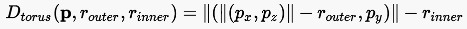``````float torus(vec3 p, float ri, float ro) {
vec2 q = vec2(length(p.xz) - ri, p.y);
return length(q) - ro;
}
``````

An example:

``````float distf(in vec3 p) {
}
``````#### Cylinder (infinite)

An infinite cylinder of radius r and going along the Y axis:``````float cylinder(in vec3 p, float r) {
return length(p.xz) - r;
}
``````
``````
float distf(in vec3 p) {
return cylinder(p, 0.2);
}
``````At first sight, it may not appear too useful as a building block. But it actually is useful, as we'll see later.

#### Cone (infinite)

Similarly, an infinite cone, with the tip at (0,0,0) and going infinitely downwards. In our case the cone is specified by a 2D vector n, which is a normal to the cone's side: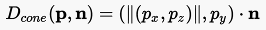``````float cone(vec3 p, vec2 n) {
return dot(vec2(length(p.xz), p.y), n);
}
``````

For example:

``````float distf(in vec3 p) {
return cone(p, normalize(vec2(1.0, 0.1)));
}
``````There are quite a few other primitives, like boxes etc (including the cool ones with rounded corners). Check the corresponding Inigo Quilez' page.

For our Christmas tree we only need the described ones, though.

### Distance function: boolean operations

The primitives on their own are not too exciting. But here comes the kicker: boolean operations on the primitives' distance fields are expressed quite easily. Which opens a world of opportunities for combining things.

#### Union

Union is the most basic operation for "adding" objects to the scene. It's a min-function (which kind of makes sense intuitively: the closest distance "wins"):``````float add(float d1, float d2) {
return min(d2, d1);
}
``````

And now we can build a bit more complex scenes, for example combine a sphere and a torus:

``````float distf(vec3 p) {
float d1 = sphere(p, 0.5);
float d2 = torus(p, 0.5, 0.3);
}
``````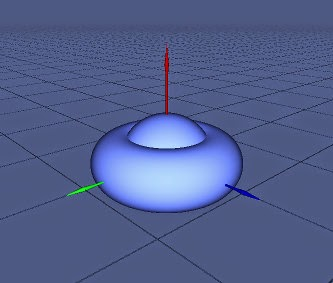#### Intersection

Intersection is just a max-function of two distances:``````float intersect(float d1, float d2) {
return max(d2, d1);
}
``````
``````float distf(vec3 p) {
float d1 = sphere(p, 0.5);
float d2 = torus(p, 0.5, 0.3);
return intersect(d1, d2);
}
``````#### Difference

Also simple, allowing for even more interesting and complex shapes: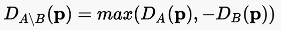``````
float diff(float d1, float d2) {
return max(-d2, d1);
}
``````
``````float distf(vec3 p) {
float d1 = sphere(p, 0.5);
float d2 = torus(p, 0.5, 0.3);
return diff(d1, d2);
}
``````#### Combined boolean operations

Now, we can mix the boolean operations to our liking. One nice trick is using the plane primitive to chop the unwanted parts off:

``````float distf(vec3 p) {
float d1 = sphere(p, 0.5);
float d2 = torus(p, 0.5, 0.3);

//  cut with a plane
float d3 = plane(p, vec3(0.0, 0.0, -1.0), -0.1);
d = diff(d, d3);

return d;
}
``````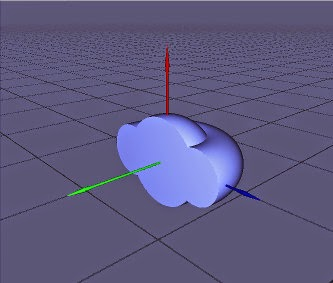### Distance function: Affine transformations

Still, having all the primitives centered at (0,0,0) is a bit boring. We want to move, rotate, scale them around. That's what the affine transformations do.

#### Translation

One important thing to note is that while we were applying boolean operations to the results of other distance functions, the affine transformations are working on their arguments instead. For example, to "move" a primitive sphere, we instead move the input point p, so that it "believes" that the sphere is still centered at (0,0,0):``````vec3 translate(vec3 p, vec3 d) {
return p - d;
}
``````

An example of translating a complex primitive:

``````
float scene(vec3 p) {
float d1 = sphere(p, 0.5);
float d2 = torus(p, 0.5, 0.3);
float d3 = plane(p, vec3(0.0, 0.0, -1.0), -0.1);
return diff(d, d3);
}

float distf(vec3 p) {
p = translate(p, vec3(1.0, 0.5, 0.0));
return scene(p);
}
``````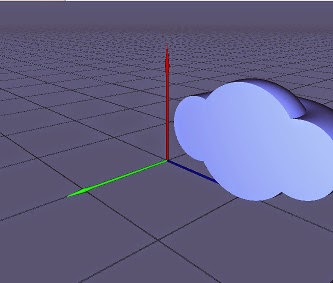#### Rotation

A rotation around Y axis (the one looking upward) is:Note that the formula does not affect the third vector component (and is not dependent on it), so in this we can write the code for a two-component vector:

``````vec2 rotate(vec2 p, float ang) {
float c = cos(ang), s = sin(ang);
return vec2(p.x*c - p.y*s, p.x*s + p.y*c);
}
``````

And an example:

``````float distf(vec3 p) {
p.xy = rotate(p.xy, PI*0.25);
return scene(p);
}
``````We can employ one little trick here and use GLSL component swizzling to rotate around different axis. For example, around X axis:

``````float distf(vec3 p) {
p.yz = rotate(p.yz, PI*0.25);
return scene(p);
}
``````A more general rotation, around an arbitrary axis, can be represented either as a sequence of rotations or via quaternions, to avoid so-called gimbal lock problem, but let's not go there.

#### Scale

Scale is different in a sense that it modifies both the argument and the result of the distance function in question:``````float distf(vec3 p) {
float scale = 3.0;
return scene(p/scale)*scale;
}
``````Intuitively, it's like Alice having a "Drink Me" potion, growing three times bigger (according to the scale value) and using her steps to measure the distance to the object. Then, having an "Eat Me" cake to grow normal again and trying to raymarch to the very object using the just measured amount of steps. Obviously, those were "big Alice" steps, so they need to be rescaled correspondingly, so that "normal Alice" could use them.

#### Order of translation/rotation/scale

It matters, so whatch out.

### Distance function: Extruding to infinity

There is more that can be done by transforming the distance function argument. One example is clamping, which essentially extrudes an object out to infinity along the given axis (here it's Y):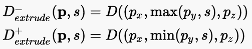Check out this infinite pencil:

``````
float distf(vec3 p) {
p.y = max(p.y, 0.0); //  extrude the base of the cone down to infinity (use min to extrude up)
p = translate(p, vec3(0.0, 1.5, 0.0)); // move the cone 1.5 units upward
float d = cone(p, normalize(vec2(1.0, 0.1))); // the infinite cone
return d;
}
``````### Distance function: swapping coordinates

The earlier described primitives are consistently oriented with "upwards" going along the Y axis. Using the GLSL vector component swizzling this can be adjusted, to orient them differently. Here, for example, we swap y and z coordinates and have the torus oriented along the Z axis instead:

``````float distf(in vec3 p) {
}
``````### Distance function: symmetry

Another useful operation is symmetric mirroring, which is achieved by just taking absolute values from the input coordinates:``````
float distf(in vec3 p) {
//  mirror upper part symmetrically about XoZ plane
//  (p.y = -abs(p.y) for the lower part)
p.y = abs(p.y);
}
``````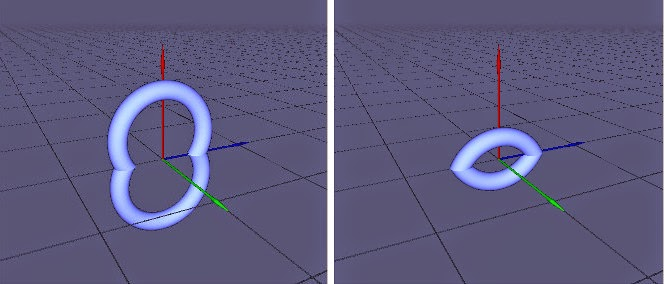### Distance function: capping infinite primitives

Now that we know the simple distance primitives and basic operations on them, we could easily derive the distance functions for things like conventional (finite) cylinder/cone:

#### Cylinder

To get a capped cylinder of height h:

• Take an infinite cylinder
• Chop it by a plane from top
• Mirror symmetrically about the ground plane``````
float capped_cylinder(in vec3 p, float r, float h) {
p.y = abs(p.y);  //  mirror upper part about XoZ plane
float cyl = cylinder(p, r);
float capPlane = plane(p, vec3(0.0, 1.0, 0.0), h*0.5);
float d = intersect(cyl, capPlane); // cap cylinder from the top
return d;
}
``````

Which can be simplified to the equivalent code:

``````float cylinder(in vec3 p, float r, float h) {
float d = cylinder(p, r);
return max(d, abs(p.y) - h*0.5);
}
``````

#### Cone

Similarly to the cylinder, to get a capped cone of height h and base radius r:

• Take an infinite cone (the normal of which needs to be computed from h and r
• Move it upwards by h
• Chop from the bottom by the XoZ plane``````float capped_cone(vec3 p, float r, float h)
{
//  find the cone normal
float ang = atan(r, h);
vec2 n = vec2(cos(ang), sin(ang));
//  move upwards by h
vec3 pt = translate(p, vec3(0.0, h, 0.0));
//  infinite cone
float infCone = cone(pt, n);
//  cap by the ground plane
float capPlane = plane(p, vec3(0.0, 1.0, 0.0), 0.0);
float d = diff(infCone, capPlane);
return d;
}
``````

Which, again, can be simplified to an equivalent code:

``````float cone(vec3 p, float r, float h) {
float ang = atan(r, h);
vec2 n = vec2(cos(ang), sin(ang));
float d = cone(vec3(p.x, p.y - h, p.z), n);
return max(d, -p.y);
}
``````

Note that we could avoid some extra computations if we passed not the height/radius pair, but rather the height/normal. That would make the function interface a bit more confusing, though.

### Distance function: Distortions

One more type of the distance field modifications is adding perturbations to the shapes. We modify the result of some distance function via the distortion function d, which is itself dependent on p: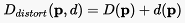It may be more clear from an example:

``````float wigglies(vec3 p) {
return cos(p.y*40.0)*0.02;
}

vec2 distf(vec3 p) {
float d = torus(p.xzy, vec2(0.5, 0.1)); // the torus
d += wigglies(p); // add wiggly horizontal distortions
return d;
}
``````The distortion function can be even more elaborated. Here's how we get a bumpy sphere:

``````float bumps(vec3 p) {
return cos(atan(p.x, p.z)*30.0)*0.01*(0.5 - p.y) + sin(p.y*60.0)*0.01;
}

vec2 distf(in vec3 p) {
float d = sphere(p, 0.5);
d += bumps(p);
return d;
}
``````One special case, the ground plane perturbed by some "noisy" distortion function, can be used to simulate a hilly terrain:

``````float ground(in vec3 p) {
return p.y + (sin(sin(p.z*0.1253) - p.x*0.311)*0.31 + cos(p.z*0.53 + sin(p.x*0.127))*0.12)*1.7 + 0.2;
}
``````Note that the actual function in the example pretty arbitrary. No doubt one could get something nicer looking with fewer instructions, after spending some time tweaking the parameters.

### Distance function: cloning the primitives

At this point you may have started wondering: "well, that's all nice and well, but are we going to add every needle in the Christmas tree, in a loop, via a boolean operation?.. in a fragment shader?.. that does not scale at all!"

That's true, it does not scale, but fortunately we have another trick up our sleeves, again thanks to the properties of the distance functions.

We can clone an object almost for free using the modulo operations. The idea is again to transform the distance function argument.

Intuitively, it's that we split the space into cells, find which cell our point p gets into and pretend that this cell has its own copy of the "world". Essentially, we manipulate with the local coordinate systems of the objects in a way similar to what we've been doing with the affine transforms, symmetrical mirroring etc.

#### Positional cloning

We can get the object infinitely cloned along some axis (let's say, X) by taking modulo and offsetting the input p.x coordinate:``````float repeat(float coord, float spacing) {
return mod(coord, spacing) - spacing*0.5;
}
``````

Let's clone a cylinder:

``````float distf(in vec3 p) {
p.x = repeat(p.x, 0.7);
return cylinder(p, 0.2, 1.0);
}
``````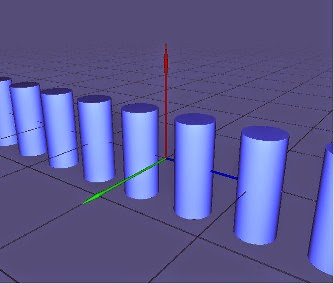Or, along both X and Z axes:

``````float distf(in vec3 p) {
p.x = repeat(p.x, 0.7);
p.z = repeat(p.z, 0.2);
return cylinder(p, 0.2, 1.0);
}
``````In practice we don't often need the infinite amount of objects, however. Fortunately, that can be limited by either clamping (extruding) the input coordinates or by chopping the resulting distance by planes:

``````float distf(in vec3 p) {
p.x = repeat(clamp(p.x, -3.0, 0.0), 1.0);
p.z = repeat(clamp(p.z, -4.0, 0.0), 1.0);
return cylinder(p, 0.2, 1.0);
}
``````#### Angular cloning

Now, even a fancier type of cloning - an angular one, which is very useful in practice, as we'll see soon:``````vec2 repeatAng(vec2 p, float n) {
float ang = 2.0*PI/n;
float sector = floor(atan(p.x, p.y)/ang + 0.5);
p = rotate(p, sector*ang);
return p;
}

vec3 repeatAngS(vec2 p, float n) {
float ang = 2.0*PI/n;
float sector = floor(atan(p.x, p.y)/ang + 0.5);
p = rotate(p, sector*ang);
return vec3(p.x, p.y, mod(sector, n));
}
``````

(the second version of the function also returns the number of the sector that p is in, which can be needed for adding further variations to the cloned objects)

``````float distf(in vec3 p) {
p.xy = repeatAng(p.xy, 3.0);
return cylinder(p, 0.2, 1.0);
}
``````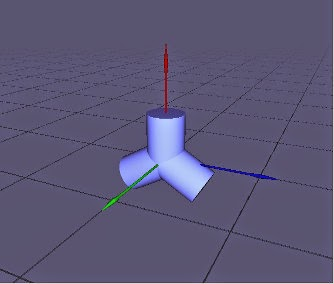repeatAng is a function from vec2 to vec2, and similarly to the rotation transform we can perform this operation around other axes by swizzling the components:

``````float distf(in vec3 p) {
p.zy = repeatAng(p.zy, 8.0);
return cylinder(p, 0.2, 1.0);
}
``````### Procedural scene description

Now that we've got a whole bunch of building blocks for our distance functions, we can finally try and describe something that we could put on a Christmas tree!

#### Materials

Before proceeding, though, we will need to make a small modification to the distance function, in order to be able to pass around not only the distance which the ray travels before hitting the scene, but also some information about the material of the point being hit. One way of doing it is make the distance function return vec2 (two-component vector) instead of float, with distance in the first component and material ID in the second:

``````vec2 distf(in vec3 p) {
vec2 obj1 = vec2(cylinder(p, 1.5, 0.1), MTL_OBJ1);
vec2 obj2 = vec2(sphere(p, 0.5), MTL_OBJ2);
return obj1;
}
``````

You may notice that boolean operations now also require to work with vec2 values instead of float, so they get modified correspondingly:

``````void diff(inout vec2 d1, in vec2 d2) {
if (-d2.x > d1.x) {
d1.x = -d2.x;
d1.y = d2.y;
}
}

void add(inout vec2 d1, in vec2 d2) {
if (d2.x < d1.x) d1 = d2;
}

void intersect(inout vec2 d1, in vec2 d2) {
if (d1.x < d2.x) d1 = d2;
}
``````

The actual material ID can be further used for shading. For example, here's how we could dispatch the diffuse material color based on the material ID:

``````vec3 getMaterialColor(float matID) {
vec3 col = BACKGROUND_COLOR;
if (matID <= MTL_OBJ1)      col = vec3(0.8, 0.8, 1.8);
else if (matID <= MTL_OBJ2) col = vec3(1.4, 1.3, 0.3);
return col;
}
``````

An interesting side-effect of performing intersection/difference of the objects with different materials is that the resulting object now has both of the materials (on the image: union, intersection, difference - left to right):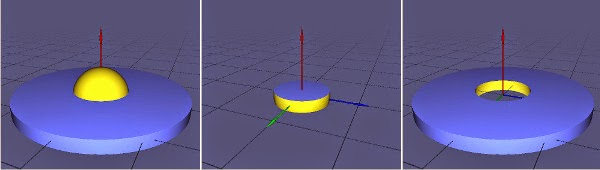#### Topper

Let's look into a little bit less obvious shapes and how to make them. For example, the Christmas tree topper, that consists of a star shape and a cylindrical holder. The code for the star looks like:

``````float star(vec3 p) {
p.xy = repeatAng(p.xy, 5.0);            // 3. Clone five cornders radially about Z axis
p.xz = abs(p.xz);                       // 2. Symmetrical about XoY and ZoY
vec3 n = vec3(0.5, 0.25, 0.8);
float d = plane(p, normalize(n), 0.1);  // 1. A plane cutting the corner of X+Y+Z+
return d;
}
``````

Note how "1 -> 2 -> 3" is kind of backwards in the code. That's because we do the most transformations on the input arguments of the current distance field. So for the star shape:

1. Have a plane primitive, that chops away the corner of the X+Y+Z+ coordinate system octant
2. Symmetrically mirror it about both XoY and ZoY planes (so we get a kind of infinite pyramid)
3. Symmetrically clone the tip of that pyramid about the Z axis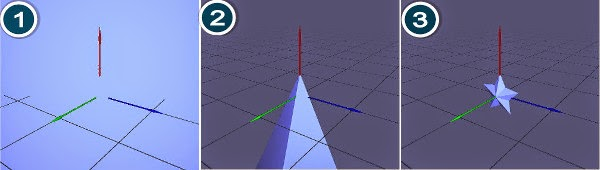Then we can scale, offset that star and add the cylindrical base:

``````#define TOPPER_SCALE 2.0
#define TOPPER_OFFSET 0.3

float topper(vec3 pos) {
pos /= TOPPER_SCALE;
pos.y -= TOPPER_OFFSET;
float base = cylinder(pos - vec3(0.0, -0.2, 0.0), vec2(0.04, 0.1));
return d;
}
``````#### Bauble

Similarly we compose other objects by using the primitives, affine transformations, boolean operations, distortions and other operations we've just looked into:

``````#define BAUBLE_SIZE 0.5
vec2 bauble(vec3 pos) {
float d = sphere(pos, BAUBLE_SIZE);
// bump
d += cos(atan(pos.x, pos.z)*30.0)*0.01*(0.5 - pos.y) + sin(pos.y*60.0)*0.01;
vec2 res = vec2(d, MTL_OBJ1);

// dent the bumped sphere
vec2 dent = vec2(sphere(pos + vec3(0.0, 0.0, -0.9), 0.7), MTL_OBJ2);
diff(res, dent);

//  the cap
pos = translate(pos, vec3(0.0, BAUBLE_SIZE + 0.03, 0.0));
float cap = cylinder(pos, BAUBLE_SIZE*0.15, 0.1);
//  the hook
cap = add(cap, torus(pos.xzy - vec3(0.0, 0.0, 0.08), BAUBLE_SIZE*0.1, 0.015));
vec2 res1 = vec2(cap, MTL_OBJ2);
return res;
}

vec2 distf(in vec3 p) {
return bauble(p);
}
``````#### Fir tree

This one is a bit more elaborated, but still uses the same principles. Let's start from making (an infinite) branch:

1. Make a single needle using a cone primitive
2. Clone that needle along the Z axis
3. Chop the half of it
4. Skew the infinite row of needles downwards, so that our needles will be eventually bent forward
5. Rotate the whole row back to align with the Z axis
7. Twist the needles to have the branch appear less regular
``````#define NEEDLE_LENGTH           0.5
#define NEEDLE_SPACING          0.2
#define NEEDLE_THICKNESS        0.05
#define NEEDLE_BEND             0.99
#define NEEDLE_TWIST            1.6
#define NEEDLE_GAIN             1.5
float needles(in vec3 p) {
p.xy = rotate(p.xy, -length(p.xz)*NEEDLE_TWIST);    // 7. twist the needles
p.yz = rotate(p.yz, -NEEDLE_BEND);                  // 5. rotate the row of needles to align back with Z axis
p.y -= p.z*NEEDLE_GAIN;                             // 4. skew the row of needles down along Y
p.z = min(p.z, 0.0);                                // 3. remove the Z+ part
p.z = repeat(p.z, NEEDLE_SPACING);                  // 2. clone the needle along Z
return cone(p, NEEDLE_THICKNESS, NEEDLE_LENGTH);    // 1. A single needle (cone)
}
``````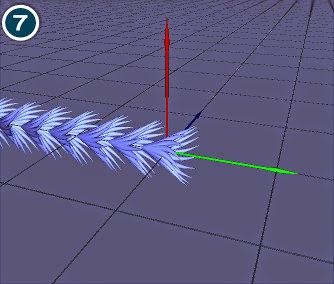There is one important problem to talk about. With all the bending and twisting going on we are distorting the space, so that distances do not get preserved anymore, and the distance function is not guaranteed to return the equal-or-less distance.

To understand why that happens, recall again Alice with her "Eat Me" cake... except this time she measures her steps using a distorted looking glass, which warps the space. After drinking the "Drink Me" potion it's not sufficient to scale the number of steps with a constant anymore: this scale will be different at every point in space.

While it may be possible to actually come up with such "inverted distortion" function for certain cases, there is an easier hack that we've already mentioned before: the step damping. Essentially, we are just being conservative and saying: "oh well, if the distance function can lie and actually penetrate the objects, we'll use only a fraction of what it returns".

Take a look at these two images. To the left is the result without step damping, while the right one has damping of 0.7:Anyway, back to our branch. Let's add a stem to it:

``````#define STEM_THICKNESS          0.05
vec2 branch(in vec3 p) {
vec2 res = vec2(needles(p), MTL_OBJ1);
float s = cylinder(p.xzy, STEM_THICKNESS);
s = intersect(s, plane(p, vec3(0.0, 0.0, 1.0), 0.0));
vec2 stem = vec2(s, MTL_OBJ2);
return res;
}
``````Now that we have a branch, we can use very similar procedure to get the whole tree:

``````#define BRANCH_ANGLE            0.5
#define BRANCH_SPACING          1.3
#define BRANCH_NUM_MAX          9.0
#define BRANCH_CURVATURE      0.08

#define TREE_H                  4.0
#define TREE_R                  3.0
#define TRUNK_WIDTH             0.025
#define TREE2_ANGLE             0.4
#define TREE2_OFFSET            0.4
#define TREE2_SCALE             0.9

vec2 halfTree(vec3 p) {
float section = floor(p.y/BRANCH_SPACING);
float numBranches =  max(2.0, BRANCH_NUM_MAX - section*BRANCH_NUM_FADE);
// 6. Revolve/clone around Y:
p.xz = repeatAng(p.xz, numBranches);
// 5. Offset to get the tree foundation:
p.z -= TREE_R;
// 4. Rotate to get the tree slope:
p.yz = rotate(p.yz, BRANCH_ANGLE);
// 3. Clone vertically:
p.y = repeat(p.y, BRANCH_SPACING);
// Offset to compensate for the bend:
p.y -= BRANCH_SPACING*0.2;
// 2. Bend it to a parabolic shape:
p.yz = rotate(p.yz, -length(p.yz)*BRANCH_CURVATURE + BRANCH_ANGLE + section*BRANCH_ANGLE_FADE);
// 1. A branch:
vec2 b =  branch(p);
return b;
}
``````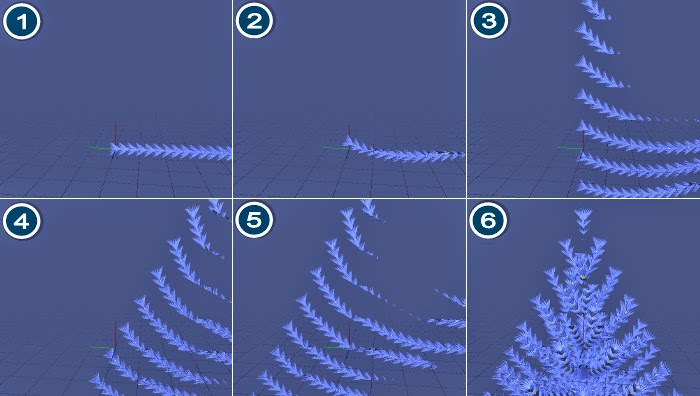There are a couple of tricks going on there with bending differently and adjusting the repeatAng factor based on the distance of the branch from the ground. Also, one can see artifacts at some intermediate stages - in this case that's OK, since we are later sweeping that part under the rag anyway.

To fake a bit more variation we repeat the "half-tree" two times, the second copy being slightly scaled, offset and rotated:

``````vec2 tree(vec3 p) {
//  the first bunch of branches
vec2 res = halfTree(p);

// the second bunch of branches (to hide the regularity)
p.xz = rotate(p.xz, TREE2_ANGLE);
p.y -= BRANCH_SPACING*TREE2_OFFSET;
p /= TREE2_SCALE;
vec2 t2 = halfTree(p);
t2.x *= TREE2_SCALE;

// trunk
vec2 tr = vec2(cone(p.xyz, TRUNK_WIDTH, TREE_H*2.0), MTL_OBJ2);

res.x = intersect(res.x, sphere(p - vec3(0.0, TREE_H*0.5 + 1.0, 0.0), TREE_H + 0.8));
return res;
}
``````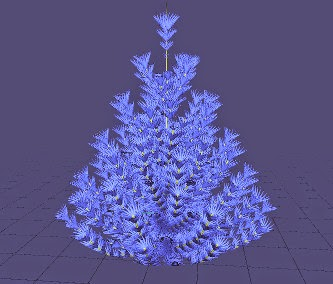It's a big topic on its own, but let's take a quick ride, especially since the actual lightning there is as basic as possible: one global directional light, Phong lighting model plus reflections and shadows.

#### Fog

Let's start from it, since it's the most obvious thing in terms of shading, which can be done with the distance value returned by the distance function. The "fog" happens by mixing in the background color exponentially, depending on the distance to the point: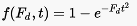``````
vec3 applyFog(vec3 col, float dist) {
return mix(col, BACKGROUND_COLOR, 1.0 - exp(-FOG_DENSITY*dist*dist));
}

vec3 render(in vec3 rayOrig, in vec3 rayDir) {
vec2 d = rayMarch(rayOrig, rayDir);
float t = d.x;
col = vec3(0.8, 0.8, 1.8); // a constant color for now
col = applyFog(col, t);
return col;
}
``````#### Materials

Seeing only the object contours is rather boring, let's try and add some colors as described in the "Materials" section. Using this, the rendering function gets modified:

``````vec3 getMaterialColor(float matID) {
vec3 col = BACKGROUND_COLOR;
if (matID <= MTL_GROUND) col = vec3(3.3, 3.3, 4.5);
else if (matID <= MTL_NEEDLE) col = vec3(0.152,0.36,0.18);
else if (matID <= MTL_STEM)   col = vec3(0.79,0.51,0.066);
else if (matID <= MTL_TOPPER) col = vec3(1.6,1.0,0.6);
else if (matID <= MTL_CAP)    col = vec3(1.2,1.0,0.8);
else                          col = jollyColor(matID);
return col;
}

vec3 render(in vec3 rayOrig, in vec3 rayDir) {
vec2 d = rayMarch(rayOrig, rayDir);
float t = d.x, matID = d.y;
col = getMaterialColor(matID); // get material color at ray the hit position
col = applyFog(col, t);
return col;
}
``````#### Lambertian (diffuse) component

This is the simplest lighting model that assumes matte surfaces. CA is the "ambient" term (which is used to fake global illumination), CL is the global directional light color. n is the surface normal at the point where it was hit by the ray, and l is the direction of light: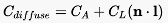The surface normal from the equation can be computed using the finite difference formula: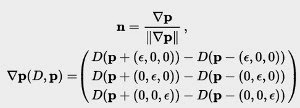``````#define NORMAL_EPS              0.001
vec3 normal(in vec3 p)
{
vec2 d = vec2(NORMAL_EPS, 0.0);
return normalize(vec3(
distf(p + d.xyy).x - distf(p - d.xyy).x,
distf(p + d.yxy).x - distf(p - d.yxy).x,
distf(p + d.yyx).x - distf(p - d.yyx).x));
}
``````

Which means that in order to compute the normal we need to evaluate the distance function six more times.

The shading code then looks like this:

``````#define GLOBAL_LIGHT_COLOR      vec3(0.8,1.0,0.9)
#define GLOBAL_LIGHT_DIR        normalize(vec3(-1.2, 0.3, -1.1))
#define AMBIENT_COLOR           vec3(0.03, 0.03, 0.03)

vec3 render(in vec3 rayOrig, in vec3 rayDir) {
vec2 d = rayMarch(rayOrig, rayDir);
float t = d.x, matID = d.y;
vec3 mtlDiffuse = getMaterialColor(mtlID);
vec3 hitPos = rayOrig + t*rayDir;
vec3 n = normal(hitPos);
float diffuse = clamp(dot(n, GLOBAL_LIGHT_DIR), 0.0, 1.0); // diffuse term
vec3 col = mtlDiffuse*(AMBIENT_COLOR + LIGHT_COLOR*diffuse);
col = applyFog(col, t);
return col;
}
``````Looks like plastic. It's fantastic!

#### Phong (specular) component

Let's bring in some shiny stuff. Phong model adds so-called specular component on top of the diffuse Lambertian one. Essentially it's a fake reflection that assumes that environment consists from a single blob light source. Here's the formula:v is view direction, alpha is the specular "sharpness" coefficient (used to adjust the shape of the reflected fake blob), r is so-called "reflection vector", which is used so commonly that GLSL has a command for it (reflect):

``````#define SPEC_POWER              16.0
#define SPEC_COLOR              vec3(0.8, 0.90, 0.60)
vec3 render(in vec3 rayOrig, in vec3 rayDir) {
vec2 d = rayMarch(rayOrig, rayDir);
float t = d.x, matID = d.y;
vec3 mtlDiffuse = getMaterialColor(mtlID);
vec3 hitPos = rayOrig + t*rayDir;
vec3 n = normal(hitPos);

// diffuse term
float diffuse = clamp(dot(n, GLOBAL_LIGHT_DIR), 0.0, 1.0);
// specular term
vec3 ref = reflect(rayDir, n);
float specular = pow(clamp(dot(ref, GLOBAL_LIGHT_DIR), 0.0, 1.0), SPEC_POWER);

vec3 col = mtlDiffuse*(AMBIENT_COLOR + LIGHT_COLOR*(diffuse + specular*SPEC_COLOR));

col = applyFog(col, t);
return col;
}
``````#### Reflections

Stil not shiny enough? Fear not, 'cause that's raytracing we are dealing with! After the ray hits an object, we can cast so called secondary rays, including the one in the direction of the reflection vector. For reflections we'll keep doing it either until we rich the hit number or raymarching step limit:

``````#define MAX_RAY_BOUNCES         3.0
#define BAUBLE_REFLECTIVITY     0.7
vec3 render(in vec3 rayOrig, in vec3 rayDir) {
vec3 resCol = vec3(0.0);
float alpha = 1.0;
for (float i = 0.0; i < MAX_RAY_BOUNCES; i++) {
vec2 d = rayMarch(rayOrig, rayDir);
float t = d.x, mtlID = d.y;
// ... SNIP ...
col = applyFog(col, t);
resCol += col*alpha; //  blend in (a possibly reflected) new color
if (mtlID <= MTL_BAUBLE ||
abs(dot(nrm, rayDir)) < 0.1) { //  poor man Fresnel
break;
}
rayOrig = pos + ref*DIST_EPSILON;
alpha *= BAUBLE_REFLECTIVITY;
rayDir = ref;
}
return vec3(clamp(resCol, 0.0, 1.0));
}
``````They are important to give depth and volume to the scene. This technique is used, again taking benefit of the fact that we are dealing with the distance fields:#### Camera

There is a piece of code that finds the primary ray direction, based on the camera position/direction and the position of the current pixel (fragment) we are raytracing:

``````vec3 getRayDir(vec3 camPos, vec3 viewDir, vec2 pixelPos) {
vec3 camRight = normalize(cross(viewDir, vec3(0.0, 1.0, 0.0)));
vec3 camUp = normalize(cross(camRight, viewDir));
return normalize(pixelPos.x*camRight + pixelPos.y*camUp + CAM_FOV_FACTOR*viewDir);
}
``````

#### Main function

Finally, the main shader function, which does the camera animation/setup, then calls the shading function to get the color of the corresponging pixel and passes it outside:

``````void main(void) {
vec2 q = gl_FragCoord.xy/iResolution.xy;
vec2 p = -1.0+2.0*q;
p.x *= iResolution.x/iResolution.y;

float ang = 0.1*(40.0 + iGlobalTime);
vec3 camPos = vec3(CAM_DIST*cos(ang), CAM_H, CAM_DIST*sin(ang));
vec3 rayDir = getRayDir(camPos,normalize(LOOK_AT - camPos), p);
vec3 color = render(camPos, rayDir);

gl_FragColor=vec4(color, 1.0);
}
``````

### Random (jolly) colors

How does one randomly pick a bauble color?

If we just "randomize" the RGB color values in a naive way, chances are that there will be a bunch of non-jolly baubles, which arguably would bring a way less joy and happiness into the house:A way of avoiding "bad" colors is to operate in some color space, where one has more control (in respect to the particular goal at hand).

For example, we can use the YIQ color space and the observation that in this space "bad" (too bleak, too dark etc) colors happen more often to the middle of the IQ plane, so we'd better avoid the middle. With some tweaking, one can achieve a "randomized" mapping from 1-dimensional value to a color scale:The correponding code:

``````
#define BAUBLE_YIQ_MUL          vec3(0.8, 1.1, 0.6)
#define BAUBLE_CLR_Y            0.7
#define BAUBLE_CLR_I            1.3
#define BAUBLE_CLR_Q            0.9

const mat3 YIQ_TO_RGB = mat3(
1.0,  0.956,  0.620,
1.0, -0.272, -0.647,
1.0, -1.108,  1.705);

vec3 jollyColor(float matID) {
vec3 clr = cos(matID*BAUBLE_YIQ_MUL);
clr= clr*vec3(0.1, BAUBLE_CLR_I, BAUBLE_CLR_Q) + vec3(BAUBLE_CLR_Y, 0.0, 0.0);
return clamp(clr*YIQ_TO_RGB, 0.0, 1.0);
}
``````

And the joy is back:Of course, a similar result could be achieved by using, for example, the HSV color space, having a constrained saturation/brighness and a random hue.

The problem in a general sense is to come up with some custom color space transformation (possibly, non-linear), and tune its parameters. The latter could be done algorithmically based on specified palette of desired (undesired) colors.

It looks jolly enough as it is, though. So let's leave the improvement as an exercise to the curious reader :)

A few words about how obfuscation of the code into the "fir tree" shape was done.

I hacked together a little script that would take the original fragment shader and first run through the C Preprocessor to get rid of all the "#define" statements:

``````cpp -P tree.frag > tree1.frag
``````

Then, Shader Minifier was used to compact the code as much as possible:

``````shader_minifier -o tree2.frag --format none tree1.frag
``````

After that, the line breaks were "creatively" inserted (manually), so the code gets the shape of three big triangles and a little rectangle at the bottom, and then a simple python one-liner was run to center all the lines to get the actual fir-tree shape.

Shader Validator is a useful tool to automatically check that the shader still compiles while doing the "creative" inserting of the line breaks:

``````glslangValidator -d tree3.frag
``````

Theoretically, it should be possible (and would be way cooler) to do the line break insertion automatically, taking, for example a template image like this: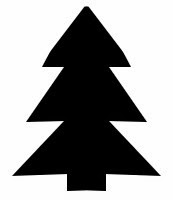as the guideline, and try to do the best at inserting line breaks such that the code still compiles and the overall code shape follows the template as close as possible. It sounds like an interesting combinatorial problem... which is left as another exercise to the reader :)

Finally, it was syntax highlighted via Pygments, using the "friendly" theme, which is conveniently green-ish:

``````python pygmentize.py -O full,style=friendly -f html -l glsl -o tree3.html tree3.frag
``````

### Final thoughts

A couple of weeks ago, I had this goal to come up with an obfuscated code that does something Christmas'y (and as a bonus, mind-boggling). Figuring out how do those magic shaders on ShaderToy work, and then writing one myself, turned out to be a suitable and rewarding challenge.

Many of the techniques, employed in those shaders, are originally coming from the computer graphics academia, and then picked up by the demoscene crowd and made into something cool-looking, just for fun.

But it often happens that those ideas, albeit not widely applicable in practice initially, eventually find their way into the mainstream. So let's wait and see how soon game engines will be using raytracing for rendering.

And even if they don't, the fundamentals of these techniques are stil useful.

One definitely interesting topic is the procedural content creation, with all its flaws and benefits. Like in the case of the Christmas tree shader, our scene is just one big math formula with a list of parameters which need to be artistically tweaked. This tweaking can be tedious, non-intuitive and frustrating (not in the small part due to the model itself being potentially flawed). Here's the screen from Fragmentarium with just a fraction of sliders:See all the pink colors?.. There it is.

Unknown said...

I notice in these functions it seems you should be returning a float but instead return a vec2. Could be a difference in parsers, but it fails on Shadertoy unless you change it to a float return value.

Thanks for all the work in putting this together!

vec2 distf(in vec3 p) {
float d = sphere(p, 0.5);
d += bumps(p);
return d;
}

wjb said...

This is one of the best distance field shader tutorials I've come across. Thanks for this and for the Distance Function Playground code as well, very helpful.

Bill

Unknown said...

Hello my fellow Americans please let show love to each others because we are all one, i suffered herpes virus many years ago, and i have been to different hospitals still yet the herpes virus spread each an everyday. one day i came across Mrs Jane from New York sharing how she was be cured from this herpes virus totally, i have know option than to contact the man called DR Peter (peterherpescurehome@gmail.com ) that help her put an end to the herpes virus within 14 days and also explain my problem to him. He also help me by preparing a native herbal medicine that flush the herpes virus from my body system and the one on my skin clear at once after using the native cream he also prepared for me. today i am happy to celebrate this coming Xmas well with my family, my advice to those that is passing through this problem don't be ashame to contact and share your problem well to Dr PETER because i also believe he can put an end to your situation within 14 days please Americans let unite together to make sure we put an end to this herpes virus please share this testimony to those that also need help and if you wanna celebrate this Xmas without any virus email him direct now: (peterherpescurehome@gmail.com)

Unknown said...

This is internet gold! I am impressed thank you!

keen said...

float sphere(vec3 p, float r) {
return length(p) - r;
}
and an example:

vec2 distf(in vec3 p) {
return sphere(p, 0.5);
}

I think here is a mistake with return type of distf

Tocci said...

Hello everyone am Ben from united states, I want to use this grate opportunity to thank Dr Afrid for the good work he has done in my life with his herbs medicine which he introduce to me, I took the herbs and everything work out well am now HIV Negative with the prove of my test result. My friends here on this website if you are facing the same problem try and get in touch with Dr Afrid herbs home via his email address drafridbalhealhome@gmail.com or WhatsApp number:+2349057260738 https//:drafridherbalhome.webs.com thanks once again Dr this man is a God fearing man and truthful

Morgan Reed said...

I want to share a testimony of how Dr UDI herbal mixture cream saved me from shame and disgrace, my penis was a big problem to me as the size was really so embarrassing ,and i was also having weak erection problem i had so many relationship called off because of my situation, i have used so many product which i found online but none could offer me the help i searched for Which was very painful and then i saw some few testimonies about this herbal specialist called Dr UDI and decided to email him on so I gave his herbal product a try. i emailed him and he got back to me and we discussed, he gave me some comforting words and encouraged me also ans then gave me his herbal pills and cream for Penis Enlargement Within 1 week of it, i began to feel the enlargement of my penis, " and now it just 2 weeks of using his products my penis is about 10 inches longer and am so happy contact Dr UDI via (drudiherbalhome@gmail.com) Via whatapp on this number +2348051243538 or you visit his website
https://drudiherba.home.blog:

Christina missy said...

My name is Christina missy. I want to share to the public how I was cured from HERPES SIMPLEX VIRUS by Dr KUTA , I visited different hospitals but they gave me list of drugs like Famvir, Zovirax, and Valtrex which is very expensive to treat the virus and never cured me. I was surfing the Internet searching for home remedies on Herpes virus and I stumbled on a page where I saw comments of people talking about how Dr KUTA helped them. So I asked for his contact, when I contacted him he gave me hope and send some Herbal medicines to me which I took for some time and it seriously worked for me, all my Herpes symptoms disappeared, I later went for a test and my Herpes result came out Negative. I am so happy and I have taking it upon myself to share this testimony, so I can save people that are going through what I went through before. My advice to you all that has lost hope already is that, there is still a chance for you, you can also get cured and live a normal and happy life again like me.
You can contact Dr KUTA directly through WhatsApp on: + 2349067603777 Or email him on: drkutaherbalcenter@gmail.com

Stanley Daniel said...

I am so happy today because I am free from herpes virus (HSV2) . I have been having herpes since the past two years now, and I have passed through many different processes in getting rid of the diseases but nothing was working out. A friend of mine introduced me to a doctor (DR Afrid drafridherbalhome@gmail.com) who sent me herbal medicines which he prepared for me, and my life got transformed and everything was fine and okay with me. Till now I have been going to check up and it is now going to four months and I am still negative.

sandra lopez said...

I have been suffering from (HERPES) disease for the last four years and had constant pain, especially in my knees. During the first year, I had faith in God that I would be healed someday.This disease started to circulate all over my body and I have been taking treatment from my doctor, a few weeks ago I came on search on the internet if I could get any information concerning the prevention of this disease, on my search I saw a testimony of someone who has been healed from (Hepatitis B and Cancer) by this Man Dr. Silver and she also gave the email address of this man and advise we should contact him for any sickness that he would be of help, so I wrote to Dr. Silver telling him about my (HERPES Virus) he told me not to worry that I was going to be cured!! hmm i never believed it,, well after all the procedures and remedy given to me by this man few weeks later I started experiencing changes all over me as the Dr. assured me that I have cured, after some time i went to my doctor to confirmed if I have been finally healed behold it was TRUE, So friends my advice is if you have such sickness or any other at all you can email Dr. Silver (drsilverhealingtemple@gmail.com) sir I am indeed grateful for the help I will forever recommend you to my friends!!! with your lovely Email Address ( drsilverhealingtemple@gmail.com or whatsapp him on +2348123877102

Tina Richard said...

I will start by saying to all that have COPD or Emphysema should please stop here and read up my story, So as you will know how to get your CURE. I had Chronic Obstructive Pulmonary Disease (COPD) for 15 years, My first symptoms were dry cough, chest tightness and shortness of breath. My first chest x-ray only showed bronchitis. Finally I went to a pulmonologist and was diagnosed with COPD, Am writing this article to appreciate the good work of General Herbal Center, that helped me get read of myCOPD/Emphysema that i have had for about 17years now, with no cure. After seeing a post of a Zom Sanchez from US on the Internet teying of how she was cured by Native Herbal Clinic prescribed remstifedies . I also decided to contact then for a cure and i purchased the medicine , because all i wanted was for me to get totally cure and to be free of it all my life. i Am happy today that they helped me and the remedies worked and i can proudly say that i am free from COPD and i am cured completely
its not worst thing to have COPD/EMPHYSEMA as a begin,you can reach Native Herbal Clinic:(nativehealthclinic@gmail.com or whatsapp +2348140073956

jorge said...

very nice work and very interesting, and very complete atte: jorge flores
..........
muy bonito trabajo y muy interesante, y muy completo atte: jorge flores